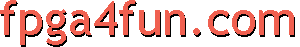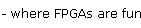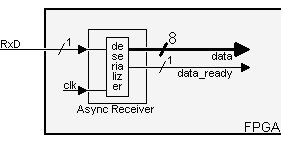## Serial interface 4 - RS-232 receiver

We are building an "async receiver":Our implementation works like that:

• The module assembles data from the RxD line as it comes.
• As a byte is being received, it appears on the "data" bus. Once a complete byte has been received, "data_ready" is asserted for one clock.

Note that "data" is valid only when "data_ready" is asserted. The rest of the time, don't use it as new data may come that shuffles it.

##### Oversampling

An asynchronous receiver has to somehow get in-sync with the incoming signal (it normally doesn't have access to the clock used by the transmitter).

• To determine when a new data byte is coming, we look for the "start" bit by oversampling the signal at a multiple of the baud rate frequency.
• Once the "start" bit is detected, we sample the line at the known baud rate to acquire the data bits.

Receivers typically oversample the incoming signal at 16 times the baud rate. We use 8 times here... For 115200 bauds, that gives a sampling rate of 921600Hz.

Let's assume that we have a "Baud8Tick" signal available, asserted 921600 times a second.

##### The design

First, the incoming "RxD" signal has no relationship with our clock.
We use two D flip-flops to oversample it, and synchronize it to our clock domain.

 reg [1:0] RxD_sync; always @(posedge clk) if(Baud8Tick) RxD_sync <= {RxD_sync, RxD};

We filter the data, so that short spikes on the RxD line aren't mistaken with start bits.

 reg [1:0] RxD_cnt; reg RxD_bit; always @(posedge clk) if(Baud8Tick) begin   if(RxD_sync && RxD_cnt!=2'b11) RxD_cnt <= RxD_cnt + 1;   else   if(~RxD_sync && RxD_cnt!=2'b00) RxD_cnt <= RxD_cnt - 1;   if(RxD_cnt==2'b00) RxD_bit <= 0;   else   if(RxD_cnt==2'b11) RxD_bit <= 1; end

A state machine allows us to go through each bit received, once a "start" is detected.

 reg [3:0] state; always @(posedge clk) if(Baud8Tick) case(state)   4'b0000: if(~RxD_bit) state <= 4'b1000; // start bit found?   4'b1000: if(next_bit) state <= 4'b1001; // bit 0   4'b1001: if(next_bit) state <= 4'b1010; // bit 1   4'b1010: if(next_bit) state <= 4'b1011; // bit 2   4'b1011: if(next_bit) state <= 4'b1100; // bit 3   4'b1100: if(next_bit) state <= 4'b1101; // bit 4   4'b1101: if(next_bit) state <= 4'b1110; // bit 5   4'b1110: if(next_bit) state <= 4'b1111; // bit 6   4'b1111: if(next_bit) state <= 4'b0001; // bit 7   4'b0001: if(next_bit) state <= 4'b0000; // stop bit   default: state <= 4'b0000; endcase

Notice that we used a "next_bit" signal, to go from bit to bit.

 reg [2:0] bit_spacing; always @(posedge clk) if(state==0)   bit_spacing <= 0; else if(Baud8Tick)   bit_spacing <= bit_spacing + 1; wire next_bit = (bit_spacing==7);

Finally a shift register collects the data bits as they come.

 reg [7:0] RxD_data; always @(posedge clk) if(Baud8Tick && next_bit && state) RxD_data <= {RxD_bit, RxD_data[7:1]};

The complete code can be found here.